## 15.2. Bar graphs

The library supports 2D vertical and horizontal bar plots as was shown in the introduction section . To use bar plots the bar plot module "`jpgraph_bar.php`" must be included in the script.

There are eight fundamental types of bar graphs supported by the library. Examples of the available types are shown in Figure 15.33

Figure 15.33. Different types of supported bar graphsa) Vertical bar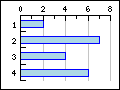b) Horizontal bar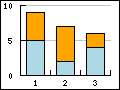c) Vertical accumulated bar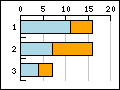d) Horizontal accumulated bare) Vertical group barf) Horizontal group barg) Vertical group accumulated barh) Horizontal group accumulated bar

Using bar plots is straightforward and works in much the same way as line plots as was discussed in the previous sections.

An instance of `class BarPlot` is created with the wanted data and is then either added directly to the graph to create a basic bar plot or is enclosed in with one of the container classes `AccBarPlot` or `GroupBarPlot` which are then added to the graph.

There is however one crucial change that is usually made. The x-scale is usually specified as a "`text`" type scale. The reasons this are primarily two

1. to get the alignment of the labels to be between the tick marks and not at the tick marks as is normal for line plots

2. to get the bars to be aligned at the center between the tick marks

The following two examples shows the difference between using an integer scale in Figure 15.34 and a text sale in Figure 15.35

 Figure 15.34. Using "int" scale for the x-axis `(example19.1.php)`Figure 15.35. Using "text" scale for the x-axis `(example19.php)`As can be seen in Figure 15.34 the bars have there left edge aligned with the data value and the tick mark (the tick mark can not be seen since it is aligned exactly with the left edge of the bar). In contrast using a text scale in Figure 15.35 adjusts the alignment of tick marks and labels in a way that is more commonly used with bar graphs.

Some other commonly used method to change the appearance of the bar graphs are

• `BarPlot::SetFillColor(\$aColor)`

This is used to specify the fill color of the bar. The argument can also be an array and in that case each color in the array is used for individual successive bars.

• `BarPlot::SetFillGradient(\$aFromColor,\$aToColor=null,\$aStyle=null)`

Specifies a gradient fill style for the bars. See ?? for details.

• `BarPlot::SetPattern(\$aPattern, \$aColor='black')`

Specifies a pattern to be used to fill the bars. See ?? for details

• `BarPlot::SetWidth(\$aWidth)`

Specifies the width of the individual bars. Of this is an integer value > 1 it is interpretated as the absolute width in pixels. If the values instead is a real number in the range [0,1] it is interpretated as the fraction of the width between the tick marks. By default the width is set to 0.4

### 15.2.1. Accumulated bar plots

Accumulated bar plots will show several data series stacked on top of each other in each bar. They are the barplot variant of accumulated area plots as was discussed previously.

An accumulated bar plot is made by aggregating one or more basic bar plots in the container class `AccBarPlot` as the following code snippet shows

 ```1 2 3 4 5 6 7 8 9 10 11 12 13 14 15 16 17 ``` ```SetFillColor('orange'); \$b2plot = new BarPlot(\$data2y); \$b2plot->SetFillColor('blue'); // Create the accumulated bar plot \$gbplot = new AccBarPlot(array(\$b1plot,\$b2plot)); // Add the accumulated plot to the graph \$graph->Add(\$gbplot); ?>```

An example of an accumulated bar plot is shown in Figure 15.36

Figure 15.36. An accumulated bar plot `(example23.php)`There are some subtleties when it comes to the formatting of the frames a round each bar in an accumulated bar plots that might be useful to know. The basic ambiguity that exists is that when we stack the bars on top of each other to create a new accumulated bar each individual bar has properties that was (or could) be set when each individual barplot was created like the frame around the plot.

For example, take the following basic accumulated bar plot (partial script)

 ```1 2 3 4 5 6 7 8 9 10 11 12 13 14 15 ``` ```SetFillGradient('AntiqueWhite2','AntiqueWhite4:0.8',GRAD_VERT); \$bplot->SetColor('darkred'); // And the second bar \$bplot2 = new BarPlot(\$datay2); \$bplot2->SetFillGradient('olivedrab1','olivedrab4',GRAD_VERT); \$bplot2->SetColor('darkgreen'); // Join them in an accumulated (stacked) plot \$accbplot = new AccBarPlot(array(\$bplot,\$bplot2)); \$graph->Add(\$accbplot); ?>```

As you can see on line 5 and on line 10 we have set the frame color differently in the two individual plots. However, the accumulated bar plot also have a frame color property (and a weight as well) so what shall we use? If we run a full example based on the lines above the result is shown in Figure 15.37 below.

Figure 15.37. Accumulated bar with individual frame colors `(accbarframeex01.php)`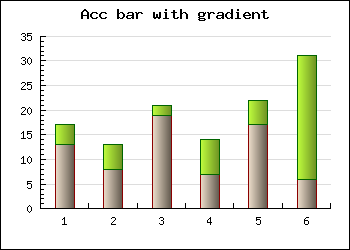As can be seen from the graph the bar around each part has the color on th frame that was set on the individual bar. There is one exception though. The line that separates the two bars are shared and will always follow the color of the top bar.

If we instead specify a frame for the accbar by adding the lines

 ```1 2 3 4 5 6 ``` ```SetColor('red'); \$accbplot->SetWeight(1); \$graph->Add(\$accbplot); ?>```

The result would be as is shown in Figure 15.38 and the properties of the accumulated bar takes precedence, again with one exception. The divider lines inside the bar is still controlled by the individual plot. By default the line weight on the accumulated bar is 0 which means that it will not be drawn, that is why we have to set a line weight on line 4 above.

Figure 15.38. Accumulated bar with unit frame color `(accbarframeex02.php)`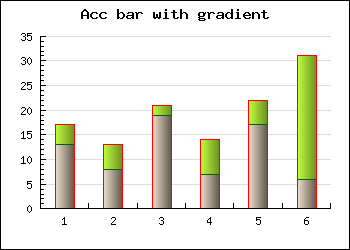If we instead were to set the individual line weight to zero, i.e.

 ```1 2 3 4 ``` ```SetWeight(0); \$bplot2->SetWeight(0); ?>```

and keep the overall frame the result would become as shown in Figure 15.39 below

Figure 15.39. Setting individual frames to weight=0 `(accbarframeex03.php)`### 15.2.2. Grouped bar plots

This uses the same principle as accumulated bar plots but instead of stacking the data series on top of each other they are shown together for the same x-value.

These types of bar graph is used to easy group two or more bars together around each tick (x-value). The bars will be placed immediately beside each other and as a group centered on each tick mark (or between if a text scale is used). A grouped bar is created by aggregating two or more ordinary bar plots and creating a `GroupBarPlot`

 ```1 2 3 4 5 6 7 8 9 10 11 12 13 14 15 16 17 18 ``` ```SetFillColor ( 'orange' ); \$b2plot = new BarPlot ( \$data2y ); \$b2plot->SetFillColor ( 'blue' ); // Create the grouped bar plot \$gbplot = new GroupBarPlot (array( \$b1plot , \$b2plot )); // Add it to the graph \$graph->Add ( \$gbplot ); ?>```

An example of this is shown in Figure 15.40

Figure 15.40. A grouped bar plot `(example21.php)`If the `SetWidth()` method is used on the `GroupBarPlot()` it will affect the total width used by all the added plots. Each individual bar width will be the same for all added bars. The default width for grouped bar is 70% of the width between the tick marks in the graph. In Figure 15.41 an example where the width is set to 90% is shown.

Figure 15.41. Adjusting the width of a group bar plot `(example22.php)`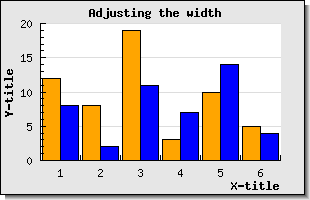The number of data points in each data series must be the same. This means that if there are no available values they should be specified as 0. An example of this is shown in Figure 15.42

Figure 15.42. All data series in a grouped bar graph must have the same number of data points `(groupbarex1.php)`### 15.2.3. Grouped accumulated bar graphs

It is perfectly possible to combine the previous bar types to have a grouped accumulated bar plot. This is done in a similar way by aggregating a number of accumulated plots in a group bar plot. The following code snippte shows how this can be done

 ```1 2 3 4 5 6 7 8 9 10 11 12 13 14 15 16 17 18 19 20 21 ``` ```SetFillColor( "orange" ); \$b2plot = new BarPlot( \$data2y ); \$b2plot->SetFillColor( "blue" ); \$b3plot = new BarPlot( \$data3y ); \$b3plot->SetFillColor( "green" ); \$b4plot = new BarPlot( \$data4y ); \$b4plot->SetFillColor( "brown" ); // Create the accumulated bar plots \$ab1plot = new AccBarPlot(array( \$b1plot , \$b2plot )); \$ab2plot = new AccBarPlot(array( \$b3plot , \$b4plot )); // Create the grouped bar plot \$gbplot = new GroupBarPlot(array( \$ab1plot , \$ab2plot )); // Add the combination to the graph \$graph->Add( \$gbplot ); ?>```

An example of this is shown in Figure 15.43 below

Figure 15.43. A grouped accumulated bar graph `(example24.php)`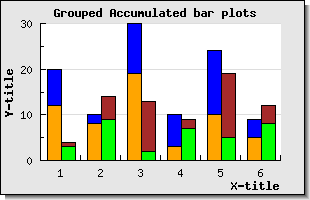### 15.2.4. Horizontal bar graphs

If a large number of values to needs to be display in a bar graph it is often better to rotated the bar graph 90 degree so that the bars are horizontal instead. There is no special graph type for this so this is achieved by rotating a standard vertical bar graph 90 degrees, usually with a call to

• `Graph::Set90AndMargin()`

The orientation of the labels of the axis will be automatically adjusted for bar graphs. A basic example is shown in Figure 15.44 below

Figure 15.44. A basic horizontal bar graph `(horizbarex1.php)`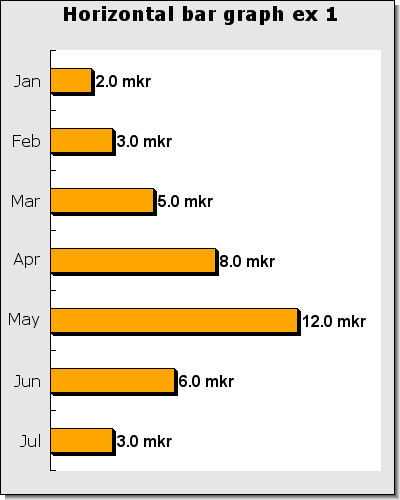The example in Figure 15.45shows how to use multiple lines as labels.

### Caution

Note that we have to use quotation marks (") and not hyphens (') in text string where we want to embed a newline character, i.e. "\n"

Figure 15.45. Using multiple line labels in a horizontal bar graph `(horizbarex4.php)`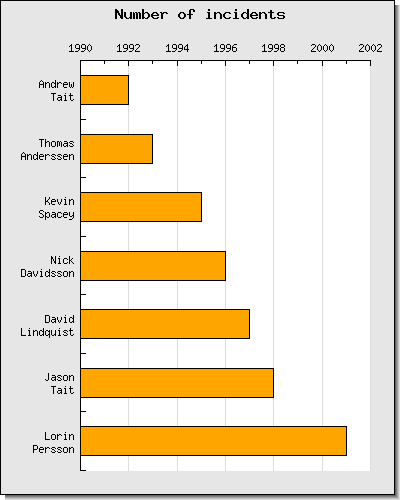### 15.2.5. Adjusting the appearance of bar graphs

#### Adjusting the width and colors

The width of each individual bar can be specified in either an absolute pixel size or as a fraction of the width between the major tick marks. The method used for this is

• `BarPlot::SetWidth(\$aWidth)`

If `\$aWidth` is an integer > 1 then it is interpretated as an absolute width in pixels. If it is a floating point number in the range [0,1] it will be interpretated as a fraction of the width between the tick marks.

It is possible to use color gradient fill for the individual bars in the bar graph.

Color gradient fill fills a rectangle with a smooth transition between two colors. In what direction the transition goes (from left to right, down and up, from the middle and out etc) is determined by the style of the gradient fill. The library currently supports 8 different styles. All supported styles are displayed in Figure 15.46 below.

Figure 15.46. Supported gradient fills for bar plots

 Figure 15.47. GRAD_MIDVER `(bargradsmallex1.php)`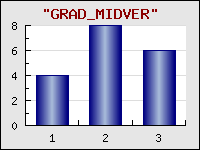Figure 15.48. GRAD_MIDHOR `(bargradsmallex2.php)`Figure 15.49. GRAD_HOR `(bargradsmallex3.php)`Figure 15.50. GRAD_VER `(bargradsmallex4.php)`Figure 15.51. GRAD_WIDE_MIDVER `(bargradsmallex5.php)`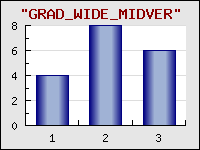Figure 15.52. GRAD_WIDE_MIDHOR `(bargradsmallex6.php)`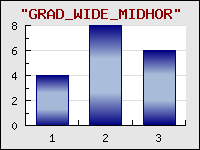Figure 15.53. GRAD_CENTER `(bargradsmallex7.php)`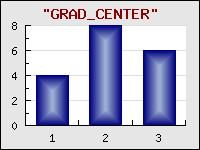Figure 15.54. GRAD_RAISED_PANEL `(bargradsmallex8.php)`To specify a gradient fill for the bar plots you make use of the method `BarPlot::SetFillGradient()` . See the class reference for details of this function.

### Caution

Gradient filling is computational expensive. Large plots with gradient fill will take in the order of 6 times longer to fill then for a normal one-color fill. This might to some extent be helped by making use of the cache feature of JpGraph so that the graph is only generated a few times.

As a final example we show an horizontal bar graph with gradient fill on both the background and the bars in Figure 15.55

Figure 15.55. Horizontal bar graph with gradient fill `(horizbarex6.php)`#### Using pattern fills

As an alternative to solid and gradient fill the bars can also have a number of patterns. This is useful for the case where black and white copies needs to be printed. The library supports the following nine different patterns as shown in Figure 15.56

Figure 15.56. Supported pattern fills for bar plots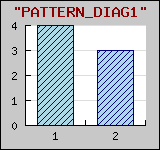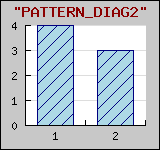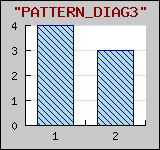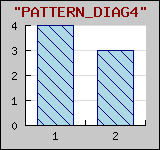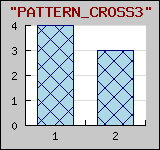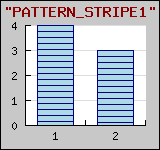To specify a pattern to be used the following method is used

• `BarPlot::SetPattern(\$aPattern,\$aColor='black')`

`\$aPattern` is one of the symbolic names as shown in Figure 15.56 If `\$aPattern` is an array then each specified pattern will be used successively for each individual bar. If there are more bars then pattern specified then pattern used will be wrapped around.

#### Displaying and formatting the value of the bar

In the same way as values could be displayed on line plots they can also be displayed on bar graphs. The formatting options for bar graphs, apart from the basic font,color and angle, also allows the specification on where on the bar the values should be displayed. This can be

• at the bottom of the bar, "`bottom`"

• at the middle of the bar, "`middle`"

• at the maximum value (but still inside the bar). "`max`"

• at the top of the bar (outside the bar), "`top`"

The position is adjusted with a call to

• `BarPlot::SetValuePos(\$aPos)`

using one of the above strings as value.

The value of the bar is enabled and controlled by accessing the "value" property of the bar plot. The following line will enable the value (this is done in exactly the same way as for line plots)

 ```1 ``` `\$barplot->value->Show();`

By default the value is displayed at the top of the bar. In the same way as for line plot it is possible to adjust the formatting of the data labels by both using a format callback function as well as statically adjusting the format, for example the angle of the label.

 ```1 2 3 4 5 6 7 8 9 10 11 12 13 ``` ```// Callback function function separator1000_usd(\$aVal) { return '\$'.number_format(\$aVal); } // Must use TTF fonts if we want text at an arbitrary angle \$bplot->value->SetFont(FF_ARIAL,FS_BOLD); \$bplot->value->SetAngle(45); \$bplot->value->SetFormatCallback('separator1000_usd'); // Black color for positive values and darkred for negative values \$bplot->value->SetColor('black','darkred'); \$graph->Add(\$bplot);```

There is one thing to take notice of here. The color of the label can be different depending on whether the bar has a positive or a negative value.

An example of using these formatting options for a bar graph is shown in Figure 15.57

Figure 15.57. Using a callback to format the labels on a bar `(barscalecallbackex1.php)`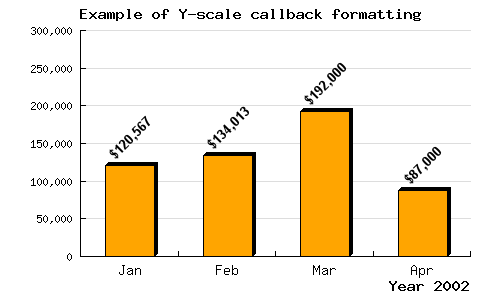• `BarPlot::SetShadow(\$aColor="black",\$aHSize=3,\$aVSize=3,\$aShow=true)`
`\$aColor`, The color of the drop shadow
`\$aHSize`, Horizontal size of the shadow
`\$aVSize`, Vertical size of the shadow
`\$aShow`, `true` = enable the shadow